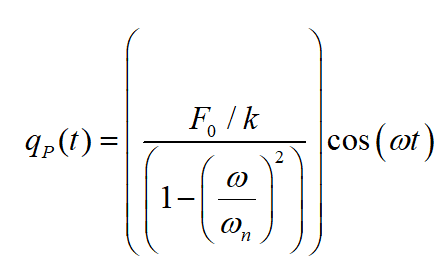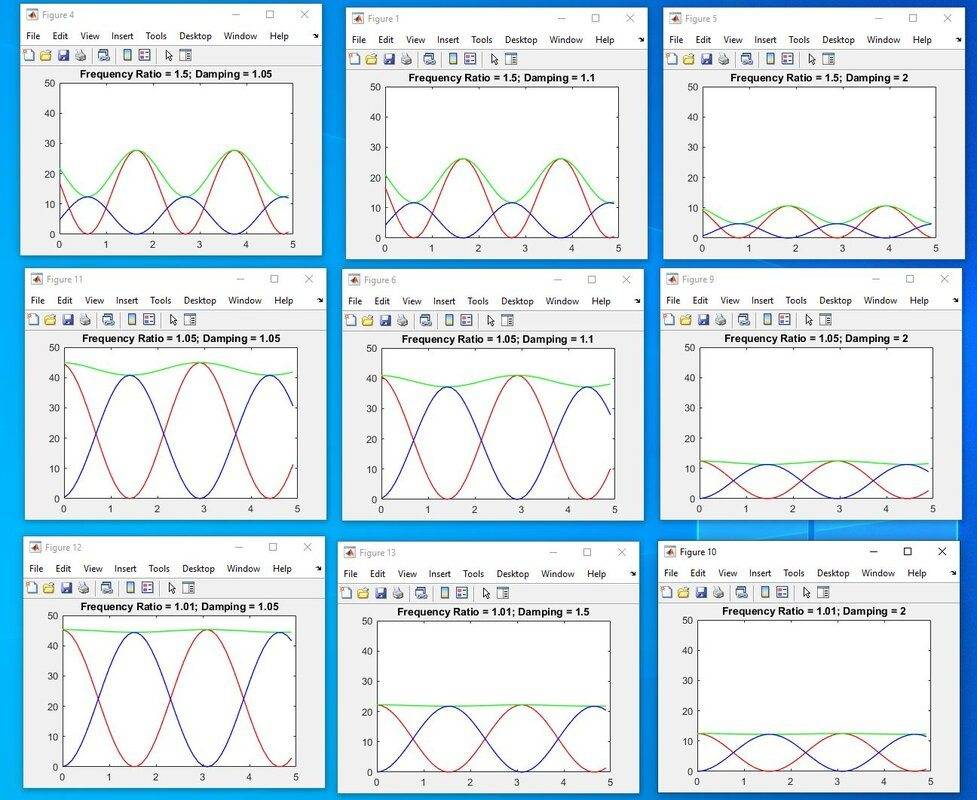# Resonance vs. Conservation of Energy

Trying2Learn
TL;DR Summary
How does resonance not violate conservation of energy?
I can solve the equation for a damped oscillator with a forcing function.

I can then plot the Kinetic and Potential Energy.

They will be out of phase, of course (KE peaking when PE is zero, and vice versa)

And we know that when the input frequency is close to the natural frequency, the system enters into resonance and "blows up" (which is sort of similar to infinite energy input (which it is not, of course).

Now I have read the following sentence on-line:

The other attribute of resonant structures is their ability to keep the energy trapped by allowing it to flow between potential and kinetic states. Resonant systems tend to trap energy.

Could someone please elaborate on this sentence, above?

KE concerns the velocity but PE contains the displacment. So how does that "trap" the little input energy¨ that I can keep inputing to the system?
How does that make the system "blow up?" As I see it, there is no "trap." The phase difference of KE and PE is simply due to the time derivative involved. And there would be a swing in this regardless of resonance. How does resonance due the "trapping?"

•Delta2

Staff Emeritus
Forget the "blow up" phrase. It's an exaggeration.

Think of the simple case of a swinging pendulum in the absence of friction. It oscillates, forever swapping K.E. for P.E. and back again. But the sum K.E.+P.E. is constant through the swing. In that sense energy is stored, and a sloppy word for stored could be trapped.

To avoid confusion, always look at the math and ignore the adjectives people use to characterize the math. Math is precise. Natural language is sloppy.

•SCP
KE concerns the velocity but PE contains the displacment. ... How does that make the system "blow up?"
Usually there are hard limits for maximal energy in KE or PE, and in case there is resonance it is possible to near the maximal values with just a small increase in each cycle.
This kind of situation rarely ends with a breakdown but in special cases this outcome is possible, and these special cases makes a good demonstration.
Hence, a good experiment 'blows up'.

•Lnewqban
Trying2Learn
Thank you everyone, however, I still have a question...

Suppose I take a 1DOF system (say spring, mass, and ignore damping) and apply an input force with a frequency close to the natural frequency. I can calculate the particular solution (ignoring homogeneous solution). For an input cosine forcing function of magnitude F0, here is the solutionk is spring constant and omegan=sqrt(k/m) is natural frequency and omega is the input frequency.

I can compute potential and kinetic energy and find that the total energy is capped.

Yet, I also have an intuitive feeling that energy is being added over time, due to the input forcing function (I cannot explain this feeling).

Also, if I look at the solution, it seems that the particular solution shows an "infinite" response immediately, upon the smallest input forcing function immediately after t=0

Here, is the solution

I understand the math.
I have a feeling for the physics.
But I cannot reconcile these issues.

The Fez
I think the misconception here is that when you are solving the mathematical equation, you are solving for the steady state, where the forcing input has been happening for an arbitrarily long time. When you are thinking about a physical situation, such as pushing a child on a swing, you are starting from zero, and your input is slowly changing the system.

Even if you had a system where the forcing frequency matched the system resonance, if you are starting from zero, there is a discontinuity at t=0 so you can't just solve the steady state equation.

Gold Member
Forget the "blow up" phrase. It's an exaggeration.
...
To avoid confusion, always look at the math and ignore the adjectives people use to characterize the math. Math is precise. Natural language is sloppy.

I disagree with your characterization. @Trying2Learn did look at the math in a subsequent post, correctly suggesting that a second-order damped harmonic oscillator will have infinite response under some conditions. Specifically, when damping is zero and ##\omega = \omega_0##. The response can, meanwhile, be very large when damping is small.

But I digress...

And we know that when the input frequency is close to the natural frequency, the system enters into resonance and "blows up" (which is sort of similar to infinite energy input (which it is not, of course).

It's more similar to infinite energy output, not input. The input is still whatever forcing function you specify.

Now I have read the following sentence on-line:

The other attribute of resonant structures is their ability to keep the energy trapped by allowing it to flow between potential and kinetic states. Resonant systems tend to trap energy.

Could someone please elaborate on this sentence, above?

KE concerns the velocity but PE contains the displacment. So how does that "trap" the little input energy¨ that I can keep inputing to the system?
How does that make the system "blow up?" As I see it, there is no "trap." The phase difference of KE and PE is simply due to the time derivative involved. And there would be a swing in this regardless of resonance. How does resonance due the "trapping?"

Yet, I also have an intuitive feeling that energy is being added over time, due to the input forcing function (I cannot explain this feeling).

Also, if I look at the solution, it seems that the particular solution shows an "infinite" response immediately, upon the smallest input forcing function immediately after t=0

I think what they are trying to express is that, in a damped harmonic oscillator, the forcing function is a constant source of new energy into the system, but the damping in the oscillator is constantly dissipating energy. If the dissipation is slower (initially) than the rate the forcing is adding energy, then some of that added energy is trapped in the system. That rate of energy dissipation is proportional to ##v^2##, so for small damping, it's going to trap some of that energy until ##v## gets large enough to dissipate energy as fast as it is being added by the forcing function. If there is no damping, the response becomes (theoretically) infinite because there is a constant energy source and no dissipation.

That infinite response is commonly referred to as "blowing up." In the real world it won't actually happen. Why? One of two things will prevent it. Real-world systems are only approximately modeled by linear ODEs like a damped harmonic oscillator. If the oscillation amplitude gets so large that the linear approximation breaks down, nonlinear effects can become large enough to be significant, while will likely include damping that didn't appear in the linear approximation. That will keep the system from going to infinity. The other option is that your system will physically break as it passes its stress limits.

•SCP, Trying2Learn, Lnewqban and 1 other person
Staff Emeritus
In the real world it won't actually happen.

Things that can't go on forever...don't.

•anorlunda
Trying2Learn
May I continue this?

I am attaching some plots for the energy of a 1DOF mass/damping/spring system with harmonic input forcing function. The system starts from rest and I am ignoring transient response. I assume simple values for mass, damping and spring constant.

• The red line is the kinetic energy (inertial)
• The blue line is the potential energy (spring)
• The green line is the sum of both of the above

In all cases, the total sum is not constant because of the harmonic forcing function that is constantly inputting energy.
1. The first row has an input frequency ratio of 1.5 * natural frequency. As you move across the row, the damping increases.
2. The second row has an input frequency of 1.05 times the natural frequency. Damping increases to the right.
3. The third row has an in put frequency of 1.01 (approaching resonace) times the natural frequency.
So we can see that in all cases, damping extracts energy

However, it seems that as we approach the natural frequency, the sum of the kinetic and potential starts to be constant and impervious to the input variation.

Can someone discuss this? I can now accept that I should not be talking about "the system blows up."
But could someone discuss in words what is happening so I can get a more intuitive understanding?

I accept that in real system, approaching resonance with no damping, some assumptions (say the linear behavior of the spring, dashpot, etc) starts to break down.

Still... what is hapening?Last edited: